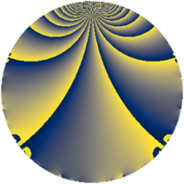# Properties

 Label 805.2.aLevel $805$ Weight $2$ Character orbit 805.a Rep. character $\chi_{805}(1,\cdot)$ Character field $\Q$ Dimension $43$ Newform subspaces $13$ Sturm bound $192$ Trace bound $11$

# Related objects

## Defining parameters

 Level: $$N$$ $$=$$ $$805 = 5 \cdot 7 \cdot 23$$ Weight: $$k$$ $$=$$ $$2$$ Character orbit: $$[\chi]$$ $$=$$ 805.a (trivial) Character field: $$\Q$$ Newform subspaces: $$13$$ Sturm bound: $$192$$ Trace bound: $$11$$ Distinguishing $$T_p$$: $$2$$, $$3$$, $$11$$

## Dimensions

The following table gives the dimensions of various subspaces of $$M_{2}(\Gamma_0(805))$$.

Total New Old
Modular forms 100 43 57
Cusp forms 93 43 50
Eisenstein series 7 0 7

The following table gives the dimensions of the cuspidal new subspaces with specified eigenvalues for the Atkin-Lehner operators and the Fricke involution.

$$5$$$$7$$$$23$$FrickeDim
$$+$$$$+$$$$+$$$+$$$4$$
$$+$$$$+$$$$-$$$-$$$9$$
$$+$$$$-$$$$+$$$-$$$4$$
$$+$$$$-$$$$-$$$+$$$5$$
$$-$$$$+$$$$+$$$-$$$4$$
$$-$$$$+$$$$-$$$+$$$5$$
$$-$$$$-$$$$+$$$+$$$4$$
$$-$$$$-$$$$-$$$-$$$8$$
Plus space$$+$$$$18$$
Minus space$$-$$$$25$$

## Trace form

 $$43 q + q^{2} + 4 q^{3} + 37 q^{4} - q^{5} + 8 q^{6} - q^{7} + 9 q^{8} + 59 q^{9} + O(q^{10})$$ $$43 q + q^{2} + 4 q^{3} + 37 q^{4} - q^{5} + 8 q^{6} - q^{7} + 9 q^{8} + 59 q^{9} - 3 q^{10} + 8 q^{12} - 14 q^{13} - 3 q^{14} + 13 q^{16} + 6 q^{17} - 7 q^{18} + 4 q^{19} - 7 q^{20} - 12 q^{22} + 11 q^{23} + 20 q^{24} + 43 q^{25} + 2 q^{26} + 16 q^{27} - 7 q^{28} - 26 q^{29} + 20 q^{30} - 16 q^{31} + 29 q^{32} + 48 q^{33} + 10 q^{34} + 7 q^{35} + 69 q^{36} - 22 q^{37} - 12 q^{38} - 12 q^{39} - 15 q^{40} + 22 q^{41} - 12 q^{42} + 4 q^{43} + 28 q^{44} - 13 q^{45} - 3 q^{46} - 40 q^{47} - 32 q^{48} + 43 q^{49} + q^{50} - 20 q^{51} - 22 q^{52} - 46 q^{53} - 20 q^{54} - 12 q^{55} - 15 q^{56} - 46 q^{58} - 12 q^{59} - 12 q^{60} - 38 q^{61} - 28 q^{62} - 13 q^{63} - 47 q^{64} - 10 q^{65} - 96 q^{66} - 28 q^{67} - 70 q^{68} - 4 q^{69} + q^{70} - 40 q^{71} - 75 q^{72} + 14 q^{73} - 50 q^{74} + 4 q^{75} + 12 q^{76} + 4 q^{77} - 28 q^{78} - 28 q^{79} + q^{80} + 131 q^{81} + 46 q^{82} - 20 q^{83} - 12 q^{84} + 2 q^{85} - 68 q^{86} + 24 q^{87} + 12 q^{88} - 10 q^{89} - 39 q^{90} - 2 q^{91} + 17 q^{92} + 40 q^{93} - 12 q^{94} + 20 q^{95} + 40 q^{96} + 14 q^{97} + q^{98} + 20 q^{99} + O(q^{100})$$

## Decomposition of $$S_{2}^{\mathrm{new}}(\Gamma_0(805))$$ into newform subspaces

Label Dim $A$ Field CM Traces A-L signs $q$-expansion
$a_{2}$ $a_{3}$ $a_{5}$ $a_{7}$ 5 7 23
805.2.a.a $1$ $6.428$ $$\Q$$ None $$-1$$ $$0$$ $$-1$$ $$-1$$ $+$ $+$ $-$ $$q-q^{2}-q^{4}-q^{5}-q^{7}+3q^{8}-3q^{9}+\cdots$$
805.2.a.b $1$ $6.428$ $$\Q$$ None $$-1$$ $$0$$ $$-1$$ $$-1$$ $+$ $+$ $-$ $$q-q^{2}-q^{4}-q^{5}-q^{7}+3q^{8}-3q^{9}+\cdots$$
805.2.a.c $1$ $6.428$ $$\Q$$ None $$2$$ $$-1$$ $$-1$$ $$-1$$ $+$ $+$ $+$ $$q+2q^{2}-q^{3}+2q^{4}-q^{5}-2q^{6}-q^{7}+\cdots$$
805.2.a.d $1$ $6.428$ $$\Q$$ None $$2$$ $$3$$ $$-1$$ $$-1$$ $+$ $+$ $-$ $$q+2q^{2}+3q^{3}+2q^{4}-q^{5}+6q^{6}+\cdots$$
805.2.a.e $2$ $6.428$ $$\Q(\sqrt{5})$$ None $$3$$ $$0$$ $$-2$$ $$-2$$ $+$ $+$ $-$ $$q+(1+\beta )q^{2}+(1-2\beta )q^{3}+3\beta q^{4}-q^{5}+\cdots$$
805.2.a.f $3$ $6.428$ $$\Q(\zeta_{14})^+$$ None $$-2$$ $$0$$ $$-3$$ $$-3$$ $+$ $+$ $+$ $$q+(-1-\beta _{2})q^{2}+(-1+2\beta _{1}-\beta _{2})q^{3}+\cdots$$
805.2.a.g $4$ $6.428$ 4.4.2777.1 None $$-2$$ $$-5$$ $$4$$ $$4$$ $-$ $-$ $+$ $$q+(-1+\beta _{3})q^{2}+(-1-\beta _{1})q^{3}+(1+\cdots)q^{4}+\cdots$$
805.2.a.h $4$ $6.428$ 4.4.22545.1 None $$-1$$ $$0$$ $$-4$$ $$-4$$ $+$ $+$ $-$ $$q-\beta _{2}q^{2}+(\beta _{1}+\beta _{3})q^{3}+(3+\beta _{3})q^{4}+\cdots$$
805.2.a.i $4$ $6.428$ 4.4.7537.1 None $$1$$ $$4$$ $$-4$$ $$4$$ $+$ $-$ $+$ $$q+\beta _{1}q^{2}+(1+\beta _{3})q^{3}+(1+\beta _{2})q^{4}+\cdots$$
805.2.a.j $4$ $6.428$ 4.4.2777.1 None $$3$$ $$6$$ $$4$$ $$-4$$ $-$ $+$ $+$ $$q+(1-\beta _{1})q^{2}+(1+\beta _{3})q^{3}+(1-\beta _{1}+\cdots)q^{4}+\cdots$$
805.2.a.k $5$ $6.428$ 5.5.122821.1 None $$-3$$ $$-6$$ $$5$$ $$-5$$ $-$ $+$ $-$ $$q+(-1+\beta _{1})q^{2}+(-1-\beta _{3})q^{3}+(\beta _{3}+\cdots)q^{4}+\cdots$$
805.2.a.l $5$ $6.428$ 5.5.255877.1 None $$-1$$ $$-4$$ $$-5$$ $$5$$ $+$ $-$ $-$ $$q-\beta _{1}q^{2}+(-1-\beta _{4})q^{3}+(1+\beta _{2})q^{4}+\cdots$$
805.2.a.m $8$ $6.428$ $$\mathbb{Q}[x]/(x^{8} - \cdots)$$ None $$1$$ $$7$$ $$8$$ $$8$$ $-$ $-$ $-$ $$q+\beta _{1}q^{2}+(1+\beta _{6})q^{3}+(1+\beta _{2})q^{4}+\cdots$$

## Decomposition of $$S_{2}^{\mathrm{old}}(\Gamma_0(805))$$ into lower level spaces

$$S_{2}^{\mathrm{old}}(\Gamma_0(805)) \cong$$ $$S_{2}^{\mathrm{new}}(\Gamma_0(23))$$$$^{\oplus 4}$$$$\oplus$$$$S_{2}^{\mathrm{new}}(\Gamma_0(35))$$$$^{\oplus 2}$$$$\oplus$$$$S_{2}^{\mathrm{new}}(\Gamma_0(115))$$$$^{\oplus 2}$$$$\oplus$$$$S_{2}^{\mathrm{new}}(\Gamma_0(161))$$$$^{\oplus 2}$$